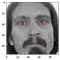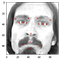# FIZZOG(Comprehensive guide): End to end Emotion detection Project

Artificial Emotional Intelligence or Emotion AI is a branch of AI that
allow computers to understand human non-verbal cues such as
body language and facial expressions.

The aim of this project is to classify people’s emotions based on their
face images by building, training and deploying a system that automatically monitors people emotions and expressions with more than 20000 facial images, with their associated facial expression labels and around 2000 images with their facial key-point annotations in it’s
dataset.

Complete code:https://github.com/astha77-bot/Fizzog.git

Part 1: We will create a deep learning model based on Convolutional
Neural Network and Residual Blocks to predict facial key-points where the dataset consists of x and y coordinates of 15 facial key points with images of 96x96 pixels.

Part 2: The second model will classify people’s emotion. Data contains images that belong to 5 categories:
0 = Angry
1 = Disgust
2 = Sad
3 = Happy
4 = Surprise

Part 3: Combine both facial key points detection and facial expression models.

Part 4: Deploy both trained models.(Deployment part of the project can be read from the follow up article.)

# Part 1: Key facial Point detection

We will create a deep learning model based on Convolutional
Neural Network and Residual Blocks to predict facial key-points where the dataset consists of x and y coordinates of 15 facial key points with images of 96x96 pixels.

Step 1:Import Datasets and Libraries

Import datasets(data.csv) and necessary libraries and make changes as required on it so as to go further with the project.

Here since the images are present in the last column as the space separated string we have to separate the values using separator and then convert it into numpy array using np.fromstring and from 1D array into 2D array of shape (96, 96).

`keyfacial_df[‘Image’] = keyfacial_df[‘Image’].apply(lambda x: np.fromstring(x, dtype = int, sep = ‘ ‘).reshape(96, 96))`

Step 2: Image visualization

We need data visualization because a visual summary of information makes it easier to identify patterns and trends than looking through thousands of rows on a spreadsheet.Therefore we’re plotting a random set of images from the dataset along with facial keypoints.

Image data is obtained from df[‘Image’] and plotted using plt.imshow where there are 15 x and y coordinates for the corresponding key facial points in the image. Since x-coordinates are in even columns like 0,2,4,.. and y-coordinates are in odd columns like 1,3,5 the range increases to the limit of 31.We access their value using .loc command, which get the values for coordinates of the image based on the column it is refering where rx represents red crosses.

`import randomfig = plt.figure(figsize=(10, 10))for i in range(64): k=random.randint(1,len(keyfacial_df)) ax = fig.add_subplot(8,8,i+1)  image = plt.imshow(keyfacial_df[‘Image’][k],cmap = ‘gray’) for j in range(1,31,2): plt.plot(keyfacial_df.loc[k][j-1], keyfacial_df.loc[k][j], ‘rx’)`

Step 3: Image Augmentation

Image augmentation is one useful technique in building convolutional neural networks that can increase the size of the training set without acquiring new images. The idea is simple; duplicate images with some kind of variation so the model can learn from more examples. Ideally, we can augment the image in a way that preserves the features key to making predictions, but rearranges the pixels enough that it adds some noise.

For those using Keras, there is a handy group of arguments in “ImageDataGenerator” that allows for image augmentation easily. The disadvantage of using Keras’ functions is that users cannot specify exactly what classes to augment, and there are limited augmentation options.

Here we will be augmenting our data by flipping the images as well as reducing the brightness and then concatenating to our dataset.

i) Flipping the images horizontally

Here we will be flipping our images horizontally, therefore Y co-ordinate would be the same and only x co-ordinate values would changes, all we have to do is to subtract our initial x-coordinate values from width of the image(here 96 is the dimension of width of image.)

`keyfacial_df_copy[‘Image’] = keyfacial_df_copy[‘Image’].apply(lambda x: np.flip(x, axis = 1))for i in range(len(columns)): if i%2 == 0: keyfacial_df_copy[columns[i]] = keyfacial_df_copy[columns[i]].apply(lambda x: 96. — float(x) )`Horizontally Flipped image

Concatenate the original data frame with the augmented data frame.

`augmented_df = np.concatenate((keyfacial_df, keyfacial_df_copy))`

ii) Increasing the brightness of images

Here we multiply pixel values by random values between 1.5 and 2 to increase the brightness of the image, we clip the value between 0 and 255 that is it should be between 0 and 255 because if the x=200 and then randomly 2 is selected then 2*200>255 which is unacceptable.

`import randomkeyfacial_df_copy = copy.copy(keyfacial_df)keyfacial_df_copy[‘Image’] = keyfacial_df_copy[‘Image’].apply(lambda x:np.clip(random.uniform(1.5, 2)* x, 0.0, 255.0))augmented_df = np.concatenate((augmented_df, keyfacial_df_copy))augmented_df.shapeplt.imshow(keyfacial_df_copy['Image'], cmap='gray')for j in range(1, 31, 2):        plt.plot(keyfacial_df_copy.loc[j-1], keyfacial_df_copy.loc[j], 'rx')`Image with increased brightness

Step 4: Data Normalization and training data preparation

The purpose of normalization is to transform data in a way that they are either dimensionless and/or have similar distributions. This process of normalization is known by other names such as standardization, feature scaling etc. Normalization is an essential step in data pre-processing in any machine learning application and model fitting.

Let’s say we have a dataset containing two variables: time traveled and distance covered. Time is measured in hours (e.g. 5, 10, 25 hours ) and speed in miles (e.g. 80, 120, 150 Kilometer/hour). Do you see the problem?

One obvious problem of course is that these two variables are measured in two different units — one in hours and the other in miles. The other problem — which is not obvious but if you take a closer look you’ll find it — is the distribution of data, which is quite different in these two variables (both within and between variables).

Therefore, Normalization gives equal weights/importance to each variable so that no single variable steers model performance in one direction just because they are bigger numbers.

`# Obtain the value of images which is present in the 31st column (since index start from 0, we refer to 31st column by 30)img = augmented_df[:,30]# Normalize the imagesimg = img/255.# Create an empty array of shape (x, 96, 96, 1) to feed the modelX = np.empty((len(img), 96, 96, 1))# Iterate through the img list and add image values to the empty array after expanding it’s dimension from (96, 96) to (96, 96, 1)for i in range(len(img)): X[i,] = np.expand_dims(img[i], axis = 2)# Convert the array type to float32X = np.asarray(X).astype(np.float32)X.shape`

(6420, 96, 96, 1)

`# Obtain the value of x & y coordinates which are to used as target.y = augmented_df[:,:30]y = np.asarray(y).astype(np.float32)y.shape`

(6420, 30)

Then split the data into train and test data.

Step 5: Understanding the neural networks, gradient descent and resnets.

Step 6: Building deep residual neural network key facial detection model

Step 7: Compiling and training key facial points detection deep learning model

`adam = tf.keras.optimizers.Adam(learning_rate = 0.0001, beta_1 = 0.9, beta_2 = 0.999, amsgrad = False)model_1_facialKeyPoints.compile(loss = “mean_squared_error”, optimizer = adam , metrics = [‘accuracy’])# Check this out for more information on Adam optimizer: https://www.tensorflow.org/api_docs/python/tf/keras/optimizers/Adam`

Step 8: Assess trained key facial points detection model performance

`with open(‘detection.json’, ‘r’) as json_file:json_savedModel= json_file.read()# load the model architecturemodel_1_facialKeyPoints = tf.keras.models.model_from_json(json_savedModel)model_1_facialKeyPoints.load_weights(‘weights_keypoint.hdf5’)adam = tf.keras.optimizers.Adam(learning_rate=0.0001, beta_1=0.9, beta_2=0.999, amsgrad=False)model_1_facialKeyPoints.compile(loss=”mean_squared_error”, optimizer= adam , metrics = [‘accuracy’])`

Evaluate the model

`result = model_1_facialKeyPoints.evaluate(X_test, y_test)print(“Accuracy : {}”.format(result))`

# Part 2: Facial Expression model

The second model will classify people’s emotion. Data contains images that belong to 5 categories:
0 = Angry
1 = Disgust
2 = Sad
3 = Happy
4 = Surprise

Step 1: Import and explore dataset for facial expression detection.

Step 2: Visualize images and plot labels.

Step 3: Perform data preparation and data augmentation.

Step 4:Build and train deep learning model for facial expression classification

Step 5:Understand how to assess classifier models(CONFUSION MATRIX, ACCURACY, PRECISION, AND RECALL)

Step 6: Assess the performance of trained facial expression classification model.

`with open(‘emotion.json’, ‘r’) as json_file:json_savedModel= json_file.read()# load the model architecturemodel_2_emotion = tf.keras.models.model_from_json(json_savedModel)model_2_emotion.load_weights(‘weights_emotions.hdf5’)model_2_emotion.compile(optimizer = “Adam”, loss = “categorical_crossentropy”, metrics = [“accuracy”])score = model_2_emotion.evaluate(X_Test, y_Test)print(‘Test Accuracy: {}’.format(score))`

Part 3: Combine both facial key points detection and facial expression models.

`def predict(X_test):# Making prediction from the keypoint modeldf_predict = model_1_facialKeyPoints.predict(X_test)# Making prediction from the emotion modeldf_emotion = np.argmax(model_2_emotion.predict(X_test), axis=-1)# Reshaping array from (856,) to (856,1)df_emotion = np.expand_dims(df_emotion, axis = 1)# Converting the predictions into a dataframedf_predict = pd.DataFrame(df_predict, columns= columns)# Adding emotion into the predicted dataframedf_predict[‘emotion’] = df_emotionreturn df_predict`

# Part 4: Deploy both trained models.

(Deployment part of the project can be read from the follow up article. See you there!)

## MLearning.ai

Data Scientists must think like an artist when finding a solution

## Sign up for AI & ART

### By MLearning.ai

A weekly collection of the best news and resources on AI & ART Take a look.

By signing up, you will create a Medium account if you don’t already have one. Review our Privacy Policy for more information about our privacy practices.

Check your inbox
Medium sent you an email at to complete your subscription.

## MLearning.ai

Data Scientists must think like an artist when finding a solution, when creating a piece of code.Artists enjoy working on interesting problems, even if there is no obvious answer.

Written by

## Astha Vijayvargiya

Computer vision## MLearning.ai

Data Scientists must think like an artist when finding a solution, when creating a piece of code.Artists enjoy working on interesting problems, even if there is no obvious answer.